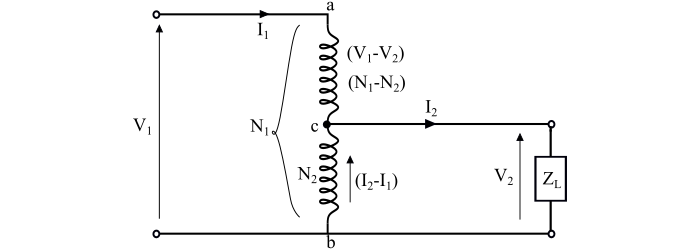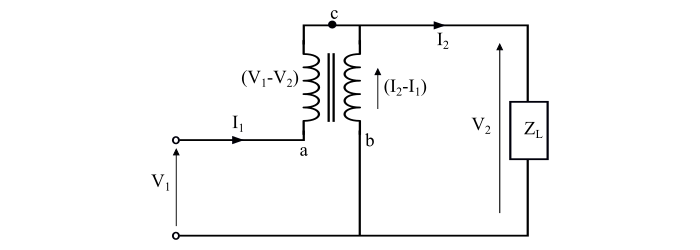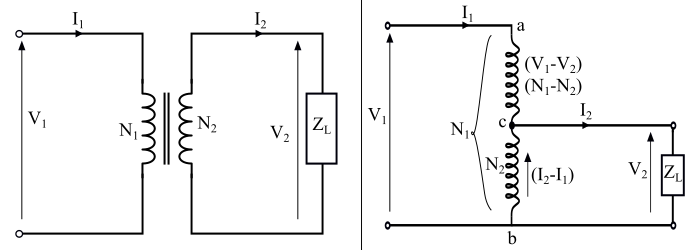# Output of an Autotransformer and Saving of Copper in Transformer

## Working of Autotransformer

The connection diagram of an ideal step-down autotransformer is shown in the figure. In which the winding ab is the primary winding having N1 turns and the winding bc is the secondary winding having N2 turns. Here, the current I1 is the input primary current and the current I2 is the output secondary current or load current.Now, the number of turns in the 'ac' portion of the winding is N1 – N2 turns and the voltage across this portion is V1 – V2. The current in the common portion (winding 'bc') of the winding is I2 – I1.

Consider the equivalent circuit of the autotransformer as shown in the figure. From this equivalent circuit, we get,$$\mathrm{\frac{𝑉_{2}}{𝑉_{1}\:−\:𝑉_{2}}=\frac{𝑁_{2}}{𝑁_{1}\:−\:𝑁_{2}}}$$

$$\mathrm{\Rightarrow\:𝑁_{1}𝑉_{2}\:−\:𝑁_{2}𝑉_{2} = 𝑁_{2}𝑉_{1}\:−\:𝑁_{2}𝑉_{2}}$$

$$\mathrm{\Rightarrow\:𝑁_{1}𝑉_{2} = 𝑁_{2}𝑉_{1}}$$

$$\mathrm{\Rightarrow\:\frac{𝑉_{2}}{𝑉_{1}}=\frac{𝑁_{2}}{𝑁_{1}}= 𝑎_{𝐴}… (1)}$$

The equation (1) is known as the voltage transformation ratio of autotransformer.

Also, from the equivalent circuit of the autotransformer, we have,

$$\mathrm{(𝑉_{1}\:−\:𝑉_{2})𝐼_{1}= 𝑉_{2}(𝐼_{2}\:−\:𝐼_{1})}$$

$$\mathrm{\Rightarrow\:𝑉_{1}𝐼_{1}\:−\:𝑉_{2}𝐼_{1} = 𝑉_{2}𝐼_{2}\:−\:𝑉_{2}𝐼_{1}}$$

$$\mathrm{\Rightarrow\:𝑉_{1}𝐼_{1}\:=\:𝑉_{2}𝐼_{2}}$$

$$\mathrm{\Rightarrow\:\frac{𝑉_{2}}{𝑉_{1}}=\frac{𝐼_{1}}{𝐼_{2}}… (2)}$$

From the eqns. (1) and (2), we get,

$$\mathrm{\frac{𝑉_{2}}{𝑉_{1}}=\frac{𝑁_{2}}{𝑁_{1}}=\frac{𝐼_{1}}{𝐼_{2}}= 𝑎_{𝐴}… (3)}$$

As the given autotransformer is ideal one, hence,

$$\mathrm{𝑉_{1}𝐼_{1}\:=\:𝑉_{2}𝐼_{2}… (4)}$$

$$\mathrm{\Rightarrow\: Input\:apparent\:power =\:Output\:apparent\:power}$$

## Output of an Autotransformer

As the primary and secondary winding of an autotransformer are connected magnetically as well as electrically. Therefore, the power from primary side is transferred to secondary side magnetically (inductively) as well as conductively.

Here,

$$\mathrm{Output\:apparent\:power = 𝑉_{2}𝐼_{2}}$$

$$\mathrm{Power\:transferred\:inductively\:= \:𝑉_{2}(𝐼_{2}\:−\:𝐼_{1})}$$

$$\mathrm{∵\:𝐼_{1}\:=\:𝑎_{𝐴}𝐼_{2}}$$

$$\mathrm{∴\:Power\:transferred \:inductively = 𝑉_{2}(𝐼_{2}\:−\:𝑎_{𝐴}𝐼_{2}) = 𝑉_{2}𝐼_{2}(1\:−\:𝑎_{𝐴})}$$

Since,

$$\mathrm{𝑉_{1}𝐼_{1}\:=\:𝑉_{2}𝐼_{2}}$$

Therefore,

$$\mathrm{Power\:transferred\:inductively \:=\:𝑉_{1}𝐼_{1}(1\:−\:𝑎_{𝐴}) = Input\:×\:(1\:− \:𝑎_{𝐴}) … (5)}$$

The equation (5) gives the amount of power that is transferred magnetically from primary to secondary.

Now, the power transferred conductively is given by,

$$\mathrm{Power\:transferred\:conductively\:=\\: (Input\:power)\:−\:(Power\:transferred\:inductively)}$$

$$\mathrm{\Rightarrow\:Power\:transferred \:conductively = (Input)\:−\:[Input\:×\:(1\:−\:𝑎_{𝐴})]}$$

$$\mathrm{= Input\:×\:(1\:−\:(1\:−\:𝑎_{𝐴}))}$$

$$\mathrm{Power\:transferred\:conductively = Input\:×\:𝑎_{𝐴}… (6)}$$

The equation (6) gives the amount of power that is transferred electrically from primary to secondary.

## Saving of Conductor Material (Copper) in Transformer

For the same ratings, i.e., same output and same transformation ratio, an autotransformer needs less conductor material (copper) as compared to an ordinary 2-winding transformer. The figure shows a two winding transformer (left) and an autotransformer (right), both are of same ratings.The length of the copper wire required in a winding is directly proportional to number of turns in the winding and the cross-sectional area of the wire is directly proportional to the current rating. Therefore, the volume and hence the weight of the copper material required in the winding is directly proportional to the product of the current and the number of turns in the winding i.e.

$$\mathrm{Weight\:of\:copper\:material \:required\:∝\:Current × Number\:of\:turns}$$

For the 2-winding transformer −

$$\mathrm{Weight\:of\:copper\:required\:∝ \:(𝐼_{1}𝑁_{1}+ 𝐼_{2}𝑁_{2})}$$

For the autotransformer −

$$\mathrm{Weight\:of\:copper\:required\:in \:section\:ac\:∝\:𝐼_{1}(𝑁_{1} − 𝑁_{2})}$$

$$\mathrm{Weight\:of\:copper\:required\:in \:section\:bc\:∝\:(𝐼_{2} − 𝐼_{1})𝑁_{2}}$$

Therefore,

$$\mathrm{Total\:Weight\:of\:copper\:required \:∝ [𝐼_{1}(𝑁_{1} − 𝑁_{2}) + (𝐼_{2} − 𝐼_{1})𝑁_{2}]}$$

Now, comparing the weight of copper required by the autotransformer and the 2-winding transformer, we get,

$$\mathrm{\frac{Weight\:of\:cu\:required\:in \:autotransformer\:(𝑊_{𝑎})}{Weight\:of\:cu\:required\:in\:2 − winding\:transformer\:(𝑊𝑜)}\=\frac{[𝐼_{1}(𝑁_{1} − 𝑁_{2}) + (𝐼_{2} − 𝐼_{1})𝑁_{2}]}{(𝐼_{1}𝑁_{1} + 𝐼_{2}𝑁_{2})}}$$

$$\mathrm{\Rightarrow\:\frac{𝑊_{𝑎}}{𝑊_{𝑜}}=\frac{𝐼_{1}𝑁_{1} − 𝐼_{1}𝑁_{2} + 𝐼_{2}𝑁_{2} − 𝐼_{1}𝑁_{2}}{𝐼_{1}𝑁_{1} + 𝐼_{2}𝑁_{2}}=\frac{𝐼_{1}𝑁_{1} + 𝐼_{2}𝑁_{2} − 2𝐼_{1}𝑁_{2}}{𝐼_{1}𝑁_{1} + 𝐼_{2}𝑁_{2}}}$$

$$\mathrm{\Rightarrow\:\frac{𝑊_{𝑎}}{𝑊_{𝑜}}=1-\frac{2𝐼_{1}𝑁_{2}}{𝐼_{1}𝑁_{1} + 𝐼_{2}𝑁_{2}}}$$

$$\mathrm{∵\:𝐼_{2}𝑁_{2}= 𝐼_{1}𝑁_{1}}$$

$$\mathrm{\Rightarrow\:\frac{𝑊_{𝑎}}{𝑊_{𝑜}}=1-\frac{2𝐼_{1}𝑁_{2}}{2𝐼_{1}𝑁_{1}}=1-\frac{𝑁_{2}}{𝑁_{1}}= (1 − 𝑎_{𝐴})}$$

Therefore, the weight of copper material required in an autotransformer is

$$\mathrm{(Weight\:of\:cu\:required\:in\: autotransformer\:(𝑊_{𝑎})) =\ (1 − 𝑎_{𝐴}) ×(Weight\:of\:cu\:required\:in\: 2 − winding\:transformer\:(𝑊_{𝑜})) … (7)}$$

Thus, the saving of copper material in an autotransformer as compared to a 2-winding transformer is given by,

$$\mathrm{Saving\:of\:copper\:material = 𝑊_{𝑜 }− 𝑊_{𝑎} = 𝑊_{𝑜} − (1 − 𝑎_{𝐴})𝑊_{𝑜}}$$

$$\mathrm{\Rightarrow\:Saving\:of\:copper \:material = 𝑎_{𝐴} × 𝑊_{𝑜} … (8)}$$

The eq. (8) gives the value of saving of copper material in an autotransformer. It is clear that the value of 𝑎𝐴 of autotransformer is nearer to 1, the greater is the saving of copper material.